2022: SklogWiki celebrates 15 years on-line

# Difference between revisions of "Berendsen thermostat"This article is a 'stub' page, it has no, or next to no, content. It is here at the moment to help form part of the structure of SklogWiki. If you add sufficient material to this article then please remove the {{Stub-general}} template from this page.
The Berendsen thermostat is a method for controlling the temperature in a molecular dynamics simulation. The Berendsen thermostat uses a weak coupling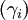$(\gamma_i)$ to an external heat bath of temperature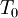$T_0$. This results in the modified equation of motion (Ref. 1 Eq. 8):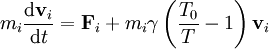$m_i \frac{{\mathrm d} {\mathbf {v}}_i}{{\mathrm d} t} = {\mathbf {F}}_i + m_i \gamma \left( \frac{T_0}{T} -1\right){\mathbf {v}}_i$.
This represents a proportional scaling of the velocities per time step from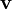${\mathbf {v}}$ to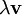$\lambda {\mathbf {v}}$, where (Ref. 1 Eq. 11)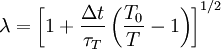$\lambda = \left[1 + \frac{\Delta t}{\tau_T} \left( \frac{T_0}{T} -1\right)\right]^{1/2}$
where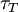$\tau_T$ is a time constant associated with the coupling.